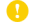Online ISSN : 1883-9029
Print ISSN : 0037-1114
ISSN-L : 0037-1114

ジャーナル フリー

1971 年 24 巻 3 号 p. 248-265[Correction] Zisin2 Vol.24 No.4 (1971) pp.e1e-e1e

Seismograms of JMA type strong motion seismograph (magnification 1.0, damping ratio 8.0 and natural period 6.0sec) have been scarcely used for a quantitative study of earthquakes because of the difficulty of actual treatment even when a good record is obtained. In this paper, a method of analysing such seismograms is proposed and tested. As an example, the seismograms of the Niigata earthquake (June 16, 1964) obtained by JMA at Tokyo was carefully analysed giving the correction of zero-line shifting, effect of a mechanical arm etc., and the ground displacement and velocity were calculated. Maximum displacement proved to be about 3cm and the velocity about 5cm/sec. Applying this ground motion to pendulums with the damping h=0.05 and the natural frequency 0.026 (0.013) 0.4cps, forced oscillation of each pendulum was calculated, and thus the response of structures with various frequencies was studied by the method of numerical simulation. By this procedure it becomes clear what kind of structure will or will not be shaken in the case of strong earthquakes and how the oscillation grows up and dies away. The maximum displacement (or velocity) was chosen from each curve and plotted as a function of natural frequency of the structure. For the periods around 5 seconds the displacement becomes maximum and is about 20cm (velocity about 20cm/sec for the period around 4 seconds). Seismic waves having the periods thereabouts are extremely important from the view point of engineering seismology, since the natural periods of many high rise buildings already constructed as well as now in blueprints are of that order.
A similar calculation was carried out for two earthquakes recorded by the DC3 strong motion seismograph at Matsushiro area in 1966, and the displacement, velocity, acceleration of the ground and the response of pendulums (structures) with various frequencies were calculated. For one of these two earthquakes, besides the above calculations, the displacement which is expected on the surface of a typical soft sediment (Toyosu, Tokyo) is calculated when the above displacement is given at the bottom of the soft layers. The surface displacement becomes several times as large as the original motion.
Acceleration spectrum of the Imperial Valley earthquake in 1940 observed at El Centro is shown together with the Niigata earthquake for reference.著者関連情報